Courses

# Test: Magnetic Lorentz Force

## 10 Questions MCQ Test Physics For JEE | Test: Magnetic Lorentz Force

Description
This mock test of Test: Magnetic Lorentz Force for JEE helps you for every JEE entrance exam. This contains 10 Multiple Choice Questions for JEE Test: Magnetic Lorentz Force (mcq) to study with solutions a complete question bank. The solved questions answers in this Test: Magnetic Lorentz Force quiz give you a good mix of easy questions and tough questions. JEE students definitely take this Test: Magnetic Lorentz Force exercise for a better result in the exam. You can find other Test: Magnetic Lorentz Force extra questions, long questions & short questions for JEE on EduRev as well by searching above.
QUESTION: 1

Solution:
QUESTION: 2

### A uniform electric field and a uniform magnetic field are produced, pointed in the same direction. An electron is projected with its velocity pointing in the same direction

Solution:

As the electron is moving along the direction of the magnetic field, it will experience no magnetic force, but due to an electric force acting on it opposite to the direction of electric field (as it is a negatively charged particle) the velocity of the electron will decreases.

QUESTION: 3

### The force acting on a charge q moving with velocity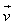in a magnetic field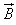is given by

Solution:

The magnetic force on a free moving charge is perpendicular to both the velocity of the charge and the magnetic field with direction given by the right hand rule . The force is given by the charge times the vector product of velocity and magnetic field.

QUESTION: 4

A Charge is fired through a magnetic field. The magnetic force acting on it is maximum when the angle between the direction of motion and magnetic field is

Solution:

The force will have a magnitude F=qvB sin q, thus it will be maximum if sin q is maximum. Thus, angle between velocity and magnetic field should be 90o or the charge particle moves perpendicular to the velocity vector.

QUESTION: 5

The frequency (v) of charged particle, moving at right angles to the magnetic field is independent of

Solution:

Frequency (v) = qB/2πm.
Frequency is independent of radius of trajectory of particle and speed of particle.

QUESTION: 6

Find the magnetic force when a charge 3.5C with flux density of 4 units is having a velocity of 2m/s.

Solution:

The magnetic force is given by F = q(v x B), where q = 3.5C, v = 2m/s and B = 4 units. Thus we get F = 3.5(2 * 4) = 28 units.

QUESTION: 7

A proton enters a magnetic field with a velocity of 1.5 x 105 m/s making an angle of 30° with the field. What is the force experienced by the proton if B = 3.0 T.​

Solution:
QUESTION: 8

When a charged particle moves in a magnetic field, its kinetic energy always

Solution:

When a charged particle enters a magnetic field B its kinetic energy remains constant as the force exerted on the particle is: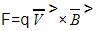is perpendicular to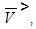so the work done by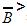=0. This does not cause any change in kinetic energy.

QUESTION: 9

A proton carrying 1 MeV kinetic energy is moving in a circular path of radius R in the uniform magnetic field. What should be the energy of an alpha particle to describe a circle of the same radius in the same field.

Solution:

Kinetic energy of a charged particle,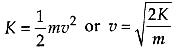Radius of the circular path of a charged particle uniform magnetic field is given by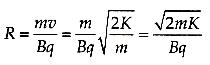Mass of a proton, mp = m

Mass of an α-particle. mα = 4m

Charge of a proton, qp = e

Charge of an α -particle, qα = 2e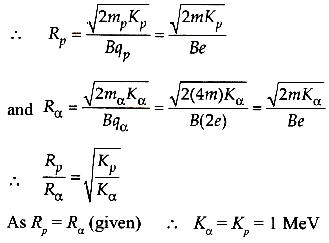QUESTION: 10

An electron is moving in a circular orbit in a magnetic field 2 x 10-3 Wb/m², the time period of electron is

Solution: